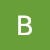# Basic Installation Guide (Windows)

Open CMD and type:

`pip install numpy`

… and done. That’s it.

# Importing NumPy

Just write down the following command:

`import numpy as np`

Here, ‘np’ is just a shorthand convention in place of numpy which is widely adopted in programming community.

# Python List Vs. NumPy Array

Python list can contain different data types within a single list, all of the elements in a NumPy array should be homogeneous. This facilitates for all the mathematical operations meant to be performed using numpy.

• NumPy arrays are faster and more compact than Python lists.
• NumPy uses much less memory to store data and it provides a mechanism of specifying the data types. This allows the code to be optimized even further.

# 1D Array, 2D Array & ND array

The NumPy `ndarray` class is used to represent both matrices and vectors.

• A vector is an array with a single dimension (there’s no difference between row and column vectors).
• A matrix refers to an array with two dimensions. For 3-D or higher dimensional arrays, the term tensor is also commonly used.

In NumPy, dimensions are called axes.

`# One dimensional array has 1 axis arr = np.array([1,2,4,5,2,3,8]) # Two dimensional array has 2 axes arr2 = np.array([[45,48,45,12],[78,15,6,25]])`

# Creating An Array In NumPy

• np.array( )

`>>> np.array([1,5,9,2]) array([1,5,9,2]`

• np.zeros( )

`>>> np.zeros(3) array([0,0,0])`

• np.ones( )

`>>> np.ones(3) array([1,1,1])`

• np.empty( )

`# Here, output is random >>> np.empty(2) array([-1.37196251e+131, -8.51127198e+092])`

• np.arange( start, end, [step] )

`>>> np.arange(2,10) array([2, 3, 4, 5, 6, 7, 8, 9]) >>> np.arange(2,10,3) array([2, 5, 8])`

• np.linspace( )

`>>> np.linspace(2,10,num = 3) array([ 2., 6., 10.])`

While the default data type is floating point (`np.float64`), you can explicitly specify which data type you want using the `dtype` keyword.

`>>> a = np.array([1,5,78.2], dtype=np.int64) >>> print(type(a)) >>> print(a) # Output : <class 'numpy.int64'> # Output : 78`

# Sorting Arrays

You can quickly sort an array in ascending order using np.sort( )

`>>> arr = np.array([12,24,5467,5]) >>> np.sort(arr) array([ 5, 12, 24, 5467])`

In addition to np.sort(), you can see all the methods that can be applied here.

You can concatenate arrays with `np.concatenate()`

`>>> a = np.array([1, 2, 3, 4, 5]) >>> b = np.array([5, 6, 7, 8]) >>> np.concatenate((a, b)) array([1, 2, 3, 4, 5, 5, 6, 7, 8])`

# Shape and Size of an Array

`>>> arr = np.array([[[0, 1, 2, 3], [4, 5, 6, 7]], [[0, 1, 2, 3], [4, 5, 6, 7]], [[0 ,1 ,2, 3], [4, 5, 6, 7]]])`

`>>> arr.ndim # Number of axis / dimension # Output : 3`

`>>> arr.size # Total number of elements in array # Output : 24`

`>>> arr.shape # Displays a tuple of integers indicating the number of elements stored along each dimension of array # Output : (3, 2, 4)`

# Reshaping an Array

Using `arr.reshape()` will give a new shape to an array without changing the data. Just remember that when you use the reshape method, the array you want to produce needs to have the same number of elements as the original array. If you start with an array with 12 elements, you’ll need to make sure that your new array also has a total of 12 elements.

`>>> a = np.array([4,8,5,6,7,2]) array([4, 8, 5, 6, 7, 2])`

`>>> a.reshape(3,2) array([[4, 8], [5, 6], [7, 2]])`

`>>> a.reshape(3,3) ValueError: cannot reshape array of size 6 into shape (3,3)`

--

--

--

## More from Bishal Shrestha

Just starting a bit.

Love podcasts or audiobooks? Learn on the go with our new app.

## Analyzing Phasewise Players in Cricket with Pandas## Copy data from one table to another table in MySQL## An Important Month Resulting In Greatness## Python vs Node.Js: Which is Best for Your Project## First steps in ASP.NET Core with Docker## How to Utilize IT Vendor Hygiene to Keep the Lights On for your Business## True Digital Transformation: Why Legacy Software Modernization Is More Than an Update## Bishal Shrestha

Just starting a bit.

## Difference between integer and string## Quick overview of random module in Python